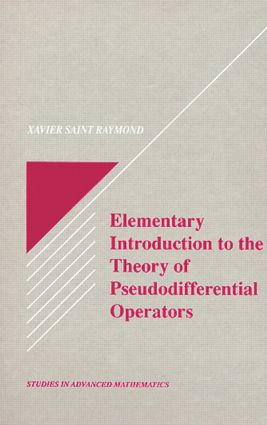Elementary Introduction to the Theory of Pseudodifferential Operators

1st Edition

CRC Press

120 pages

Purchasing Options:\$ = USD
Hardback: 9780849371585
pub: 1991-09-17
SAVE ~\$35.00
\$175.00
\$140.00
x
eBook (VitalSource) : 9780203758540
pub: 2018-02-06
from \$28.98

FREE Standard Shipping!

Description

In the 19th century, the Fourier transformation was introduced to study various problems of partial differential equations. Since 1960, this old tool has been developed into a well-organized theory called microlocal analysis that is based on the concept of the pseudo-differential operator. This book provides the fundamental knowledge non-specialists need in order to use microlocal analysis. It is strictly mathematical in the sense that it contains precise definitions, statements of theorems and complete proofs, and follows the usual method of pure mathematics. The book explains the origin of the theory (i.e., Fourier transformation), presents an elementary construcion of distribution theory, and features a careful exposition of standard pseudodifferential theory. Exercises, historical notes, and bibliographical references are included to round out this essential book for mathematics students; engineers, physicists, and mathematicians who use partial differential equations; and advanced mathematics instructors.

Fourier Transformation and Sobolev Spaces: Introduction. Functions in Rn. Fourier Transformation and Distributions in Rn. Sobolev Spaces. Exercises. Notes. Pseudodifferential Symbols: Introduction. Definition and Approximation of Symbols. Oxcillatory Integrals. Operations on Symbols. Exercises. Pseudodifferential Operators: Action in S and S'. Action in Sobolev Spaces. Invariance Under a Change of Variables. Exercises. Notes. Applications: Introduction. Local Solvability of Linear Differential Operators. Wave Front Sets of Solutions of Partial Differential Equations. The Cauchy Problem for the Wave Equation. Exercises. Notes. Index of Notations. References.